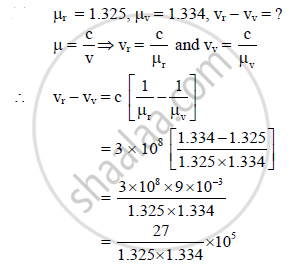HSC Science (Electronics) 12th Board ExamMaharashtra State Board
Share

# The Refractive Indices of Water for Red and Violet Colours Are 1.325 and 1.334 Respectively. Find the Difference Between the Velocities of Rays for These Two Colours in Water. - HSC Science (Electronics) 12th Board Exam - Physics

#### Question

The refractive indices of water for red and violet colours are 1.325 and 1.334 respectively.
Find the difference between the velocities of rays for these two colours in water. (c = 3 × 108 m/s)

#### Solution= [log (27) − log (1.325) − log (1.334)] × 105

= [1.4314 − 0.1222 − 0.1252] × 105

= [antilog (1.184)] × 105

= 15.28 × 105 = 1.528 × 106 m/s.

The difference between the velocities of rays for red and violet colours in water is
1.528 × 106 m/s.

Is there an error in this question or solution?

#### APPEARS IN

2012-2013 (October) (with solutions)
Question 6.3 | 3.00 marks

#### Video TutorialsVIEW ALL 

Solution The Refractive Indices of Water for Red and Violet Colours Are 1.325 and 1.334 Respectively. Find the Difference Between the Velocities of Rays for These Two Colours in Water. Concept: Polarisation.
S